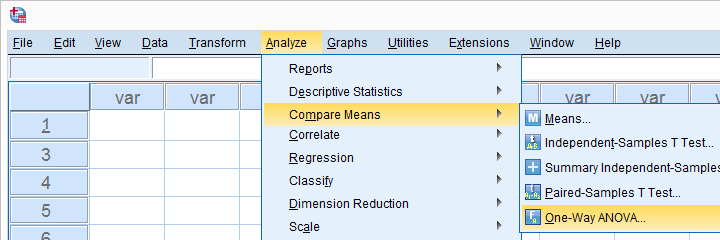SPSS TUTORIALS BASICS ANOVA REGRESSION FACTOR CORRELATION

# SPSS ANOVA Tutorials – Overview## SPSS One-Way ANOVA Tutorials

### ANOVA – Super Simple Introduction

ANOVA (analysis of variance) tests if 3+ population means are all equal.

Example: do the pupils of schools A, B and C have equal mean IQ scores?

This super simple introduction quickly walks you through the basics such as assumptions, null hypothesis and post hoc tests.

### SPSS One-Way ANOVA Tutorial

SPSS One-Way ANOVA tests whether the means on a metric variable for three or more groups of cases are all equal. The groups of cases are identified by a categorical variable.

### SPSS ANOVA with Post Hoc Tests

Post hoc tests in ANOVA test if the difference between each possible pair of means is statistically significant.

This step-by-step tutorial quickly walks you through the entire procedure.

### SPSS ANOVA without Raw Data

In SPSS, you can run a one-way ANOVA without the actual data if you have sample sizes, means and SD’s.

The output may include post-hoc tests, effect sizes and power but not Levene's test or any normality tests.

## ANOVA - Levene’s Test

### How to Run Levene’s Test in SPSS?

Levene’s test examines if 2+ populations have equal variances on some variable.

This condition -known as the homogeneity of variance assumption- is required by t-tests and ANOVA.

So how to run and interpret this test in SPSS? This simple tutorial quickly walks you through.

### SPSS ANOVA – Levene’s Test “Significant”

If Levene’s test is “Significant“ for an ANOVA, the Welch and Games-Howell tests are good alternatives.

This tutorial quickly walks you through these analyses.

## SPSS Two-Way ANOVA Tutorials

### SPSS Two-Way ANOVA with Interaction Tutorial

In ANOVA and regression, an interaction effect means that some effect depends on another variable.

Example: women become happier but men become unhappier if they have children. So the effect of having children depends on sex.

This tutorial walks you through testing for and interpreting interaction effects in ANOVA.

### SPSS Two Way ANOVA – Basics Tutorial

Run your SPSS two way ANOVA the right way in 5 simple steps. With downloadable data file, enhanced screenshots and annotated output.

## Repeated Measures ANOVA Tutorials

### Repeated Measures ANOVA – Simple Introduction

Repeated measures ANOVA tests if 3+ variables have equal means in some population.

Example: are the mean scores on IQ tests A, B and C equal for all Dutch children?

This simple introduction quickly walks you through the basics.

### SPSS Repeated Measures ANOVA Tutorial

SPSS Repeated measures ANOVA is a procedure for testing whether the means of 3 or more metric variables are equal. These variables have been measured on the same cases.

### SPSS Repeated Measures ANOVA II

This step-by-step tutorial walks you through a repeated measures ANOVA with a within and a between-subjects factor in SPSS. Covers post hoc tests and the interaction effect as well.

### SPSS RM ANOVA – 2 Within-Subjects Factors

SPSS Repeated Measures ANOVA - 2 Within-Subjects Factors

## SPSS ANOVA FAQ

### How to Get (Partial) Eta Squared from SPSS?

(Partial) eta squared is an effect size measure for one-way or factorial ANOVA. This tutorial shows 2 easy ways to get it from SPSS.

### Variance – What Is It?

The variance is a number that indicates how far a set of numbers lie apart. This tutorial explains the concept gently with examples and illustrations.

## ANCOVA - Analysis of Covariance

### SPSS ANCOVA – Beginners Tutorial

ANCOVA (analysis of covariance) tests if 2+ population means are equal while controlling for 1+ background variables.

Example: do medicines A, B and C result in equal mean blood pressures when controlling for age?

ANCOVA basically combines ANOVA and regression. This tutorial walks you through the analysis with an example in SPSS.

# Tell us what you think!

*Required field. Your comment will show up after approval from a moderator.

# THIS TUTORIAL HAS 5 COMMENTS:

•### By Laurence Moseley on May 28th, 2018

I think that your tutorials are very useful. Over the years, I have kept copies of them. When I meet people who are struggling with SPSS I point them either to the ones which I have collected, or I direct them to your website - Cheers Laurie

•### By Ruben Geert van den Berg on May 29th, 2018

Hi Laurence, thanks for the compliment!

You'd better use the online versions because we sometimes rewrite and improve some of our older tutorials -if we can find the time, that is.

•### By Robert Grunwald on October 23rd, 2018

Great SPSS ANOVA Tutorials, thank you!

•### By YOUNG on October 24th, 2019

Thank you for the useful information.
esp SPSS Repeated Measures ANOVA Tutorial

•### By Aziz Wahbi on January 13th, 2023

A lot of thanks for this great Tutorial :)